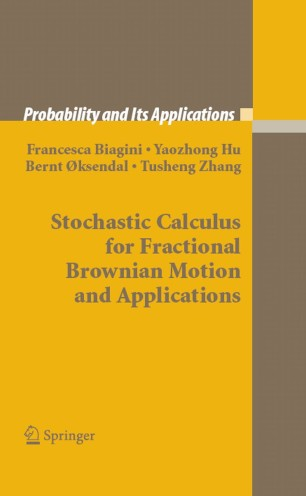# Stochastic Calculus for Fractional Brownian Motion and Applications

• Francesca Biagini
• Yaozhong Hu
• Bernt Øksendal
• Tusheng ZhangBook

Part of the Probability and Its Applications book series (PIA)

1. Front Matter
Pages i-xiv

3. ### Stochastic calculus

1. Pages 123-145
2. Pages 147-166
4. ### Applications of stochastic calculus

1. Pages 169-180
2. Pages 207-238
3. Pages 239-269
5. Back Matter
Pages 273-329

### Introduction

Fractional Brownian motion (fBm) has been widely used to model a number of phenomena in diverse fields from biology to finance. This huge range of potential applications makes fBm an interesting object of study.

fBm represents a natural one-parameter extension of classical Brownian motion therefore it is natural to ask if a stochastic calculus for fBm can be developed. This is not obvious, since fBm is neither a semimartingale (except when H = ½), nor a Markov process so the classical mathematical machineries for stochastic calculus are not available in the fBm case.

Several approaches have been used to develop the concept of stochastic calculus for fBm. The purpose of this book is to present a comprehensive account of the different definitions of stochastic integration for fBm, and to give applications of the resulting theory. Particular emphasis is placed on studying the relations between the different approaches.

Readers are assumed to be familiar with probability theory and stochastic analysis, although the mathematical techniques used in the book are thoroughly exposed and some of the necessary prerequisites, such as classical white noise theory and fractional calculus, are recalled in the appendices.

This book will be a valuable reference for graduate students and researchers in mathematics, biology, meteorology, physics, engineering and finance. Aspects of the book will also be useful in other fields where fBm can be used as a model for applications.

### Keywords

Brownian motion Markov Markov process Martingale Potential Probability theory Semimartingale Stochastic calculus calculus equation fractional Brownian motion local time mathematics model partial differential equation

#### Authors and affiliations

• Francesca Biagini
• 1
• Yaozhong Hu
• 2
• Bernt Øksendal
• 3
• Tusheng Zhang
• 4
1. 1.Mathematisches Institut80333Germany
2. 2.University of Kansas66045-2142LawrenceUSA
3. 3.University of OsloN-0316BlindernNorway
4. 4.University of ManchesterM13 9PLUK